# Kerala SSLC Board Question Papers for Class 10th Maths 2018 With Solutions In PDF

Kerala SSLC (Class 10) maths 2018 question paper with solutions are provided here in a downloadable pdf format and also in the text so that the students can easily access them. Along with the solutions, they can also get the maths question paper 2018 Class 10 SSLC for reference. Students are able to access all the Kerala board previous year maths question papers here. These can be downloaded easily and students can practice and verify the answers provided by BYJU’S. Solving 2018 Maths question papers for class 10 will help the students to predict what type of questions will appear in the exam.

### QUESTION PAPER CODE S 1827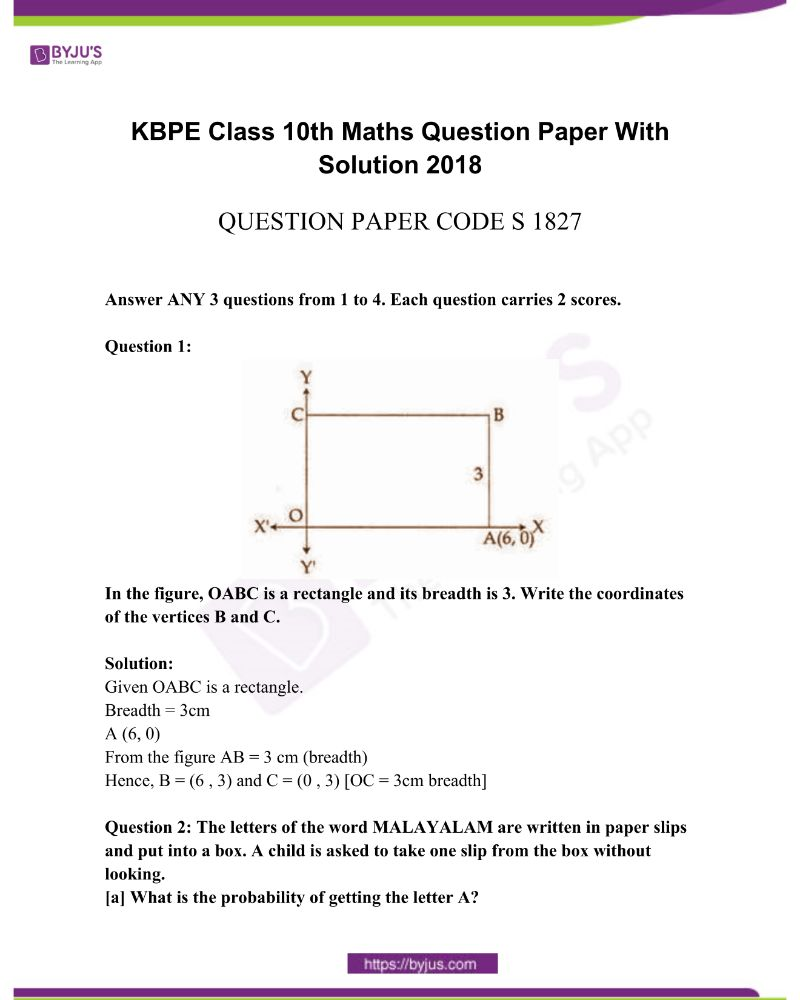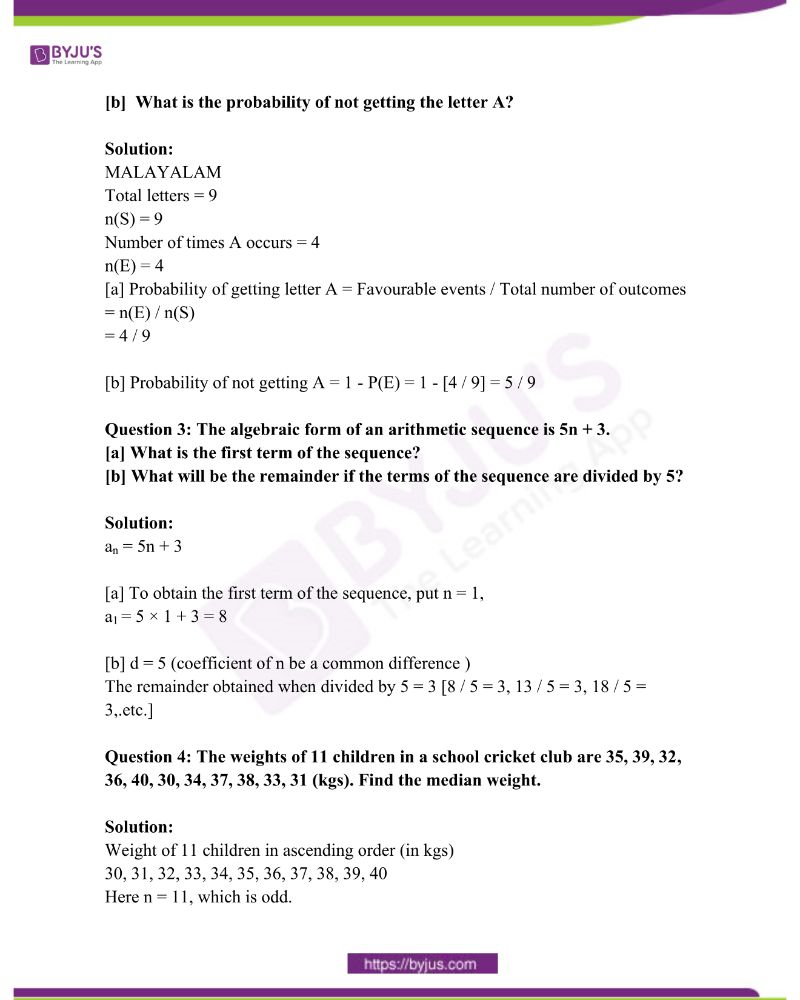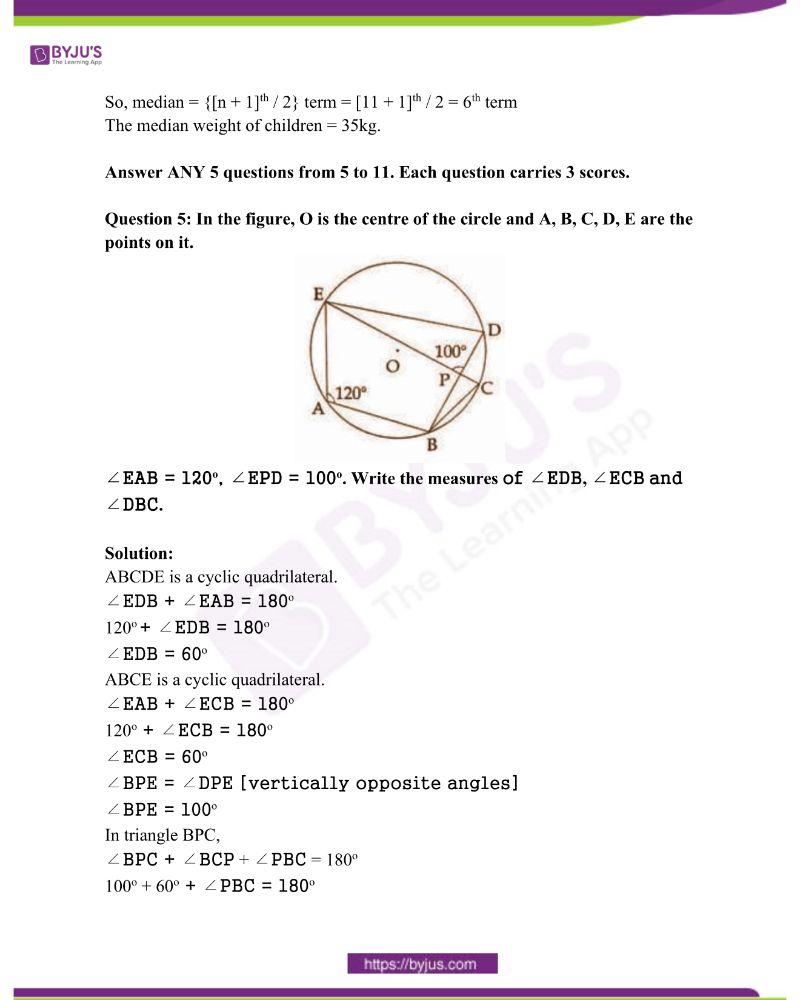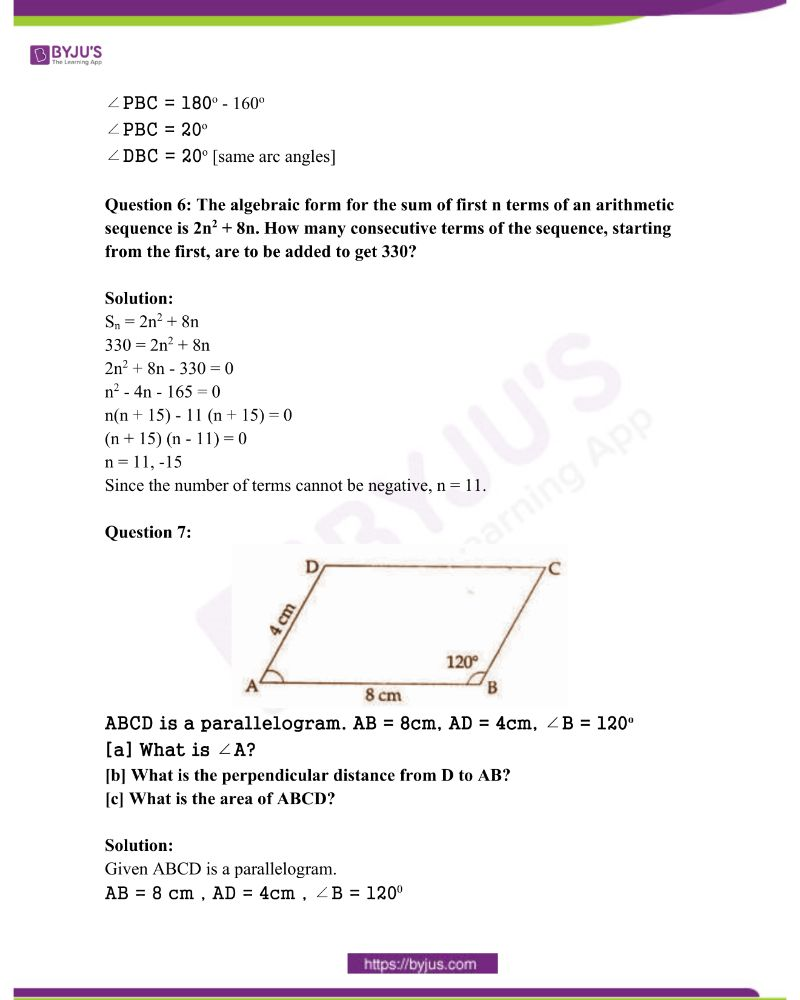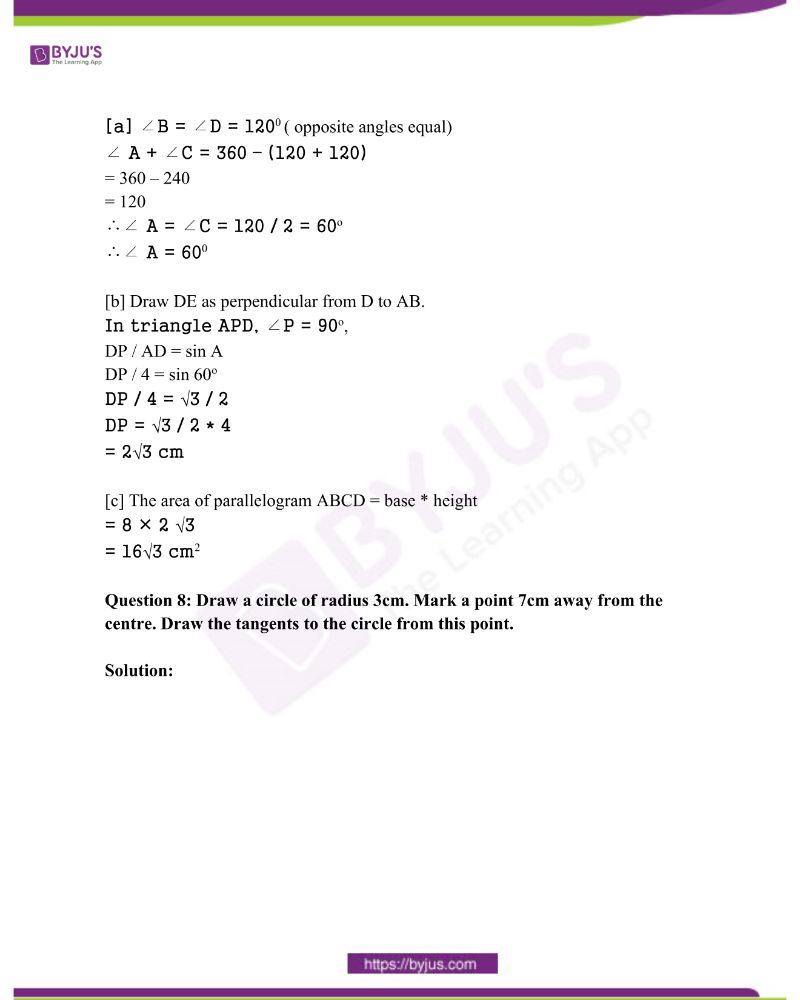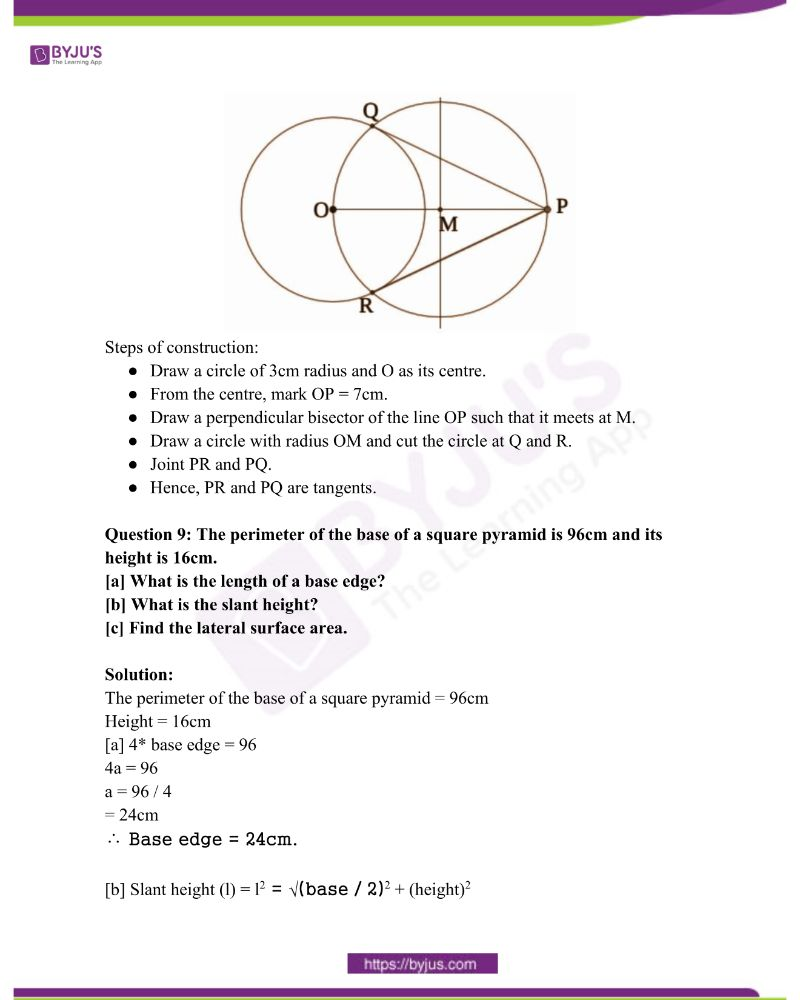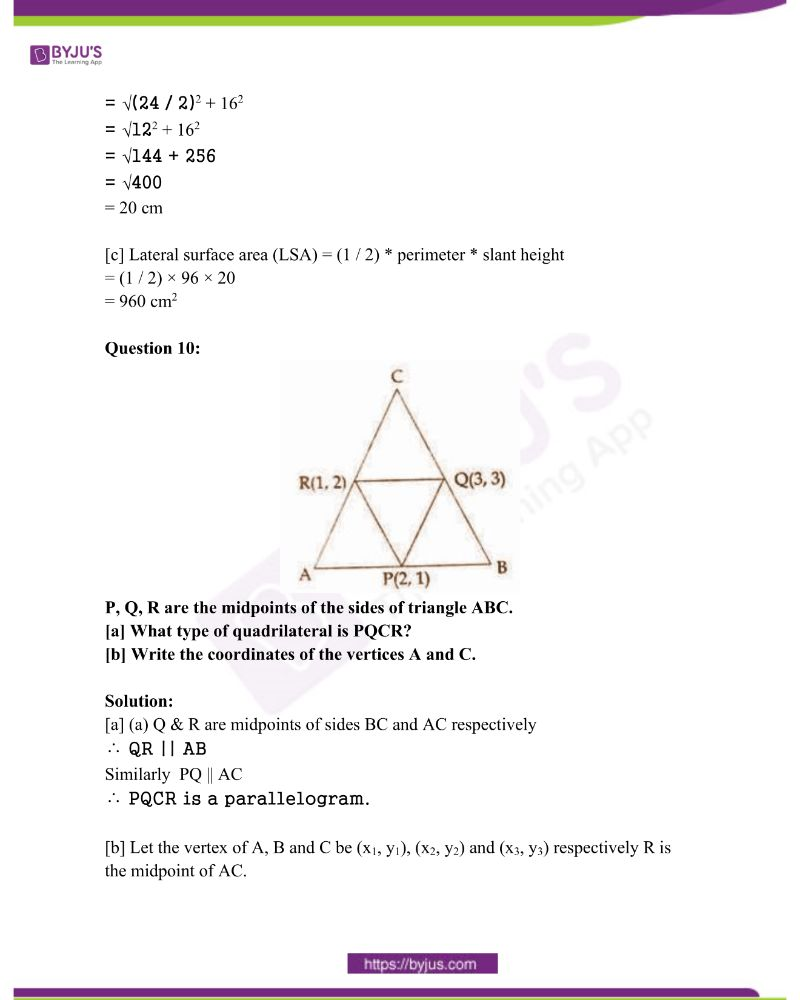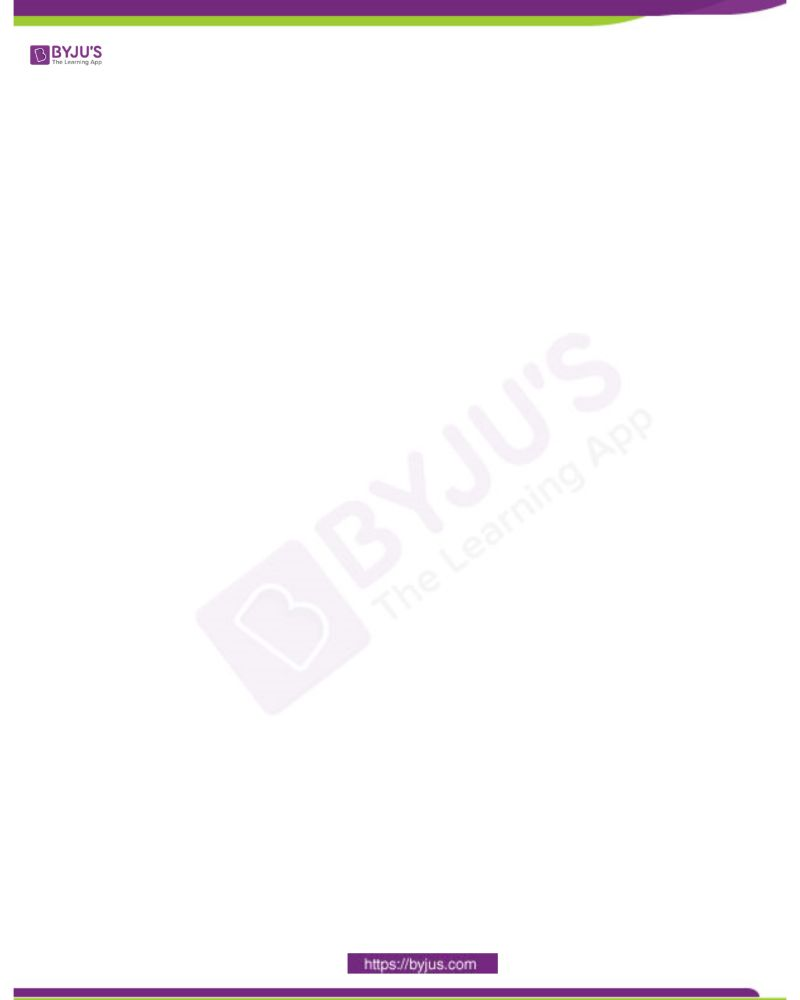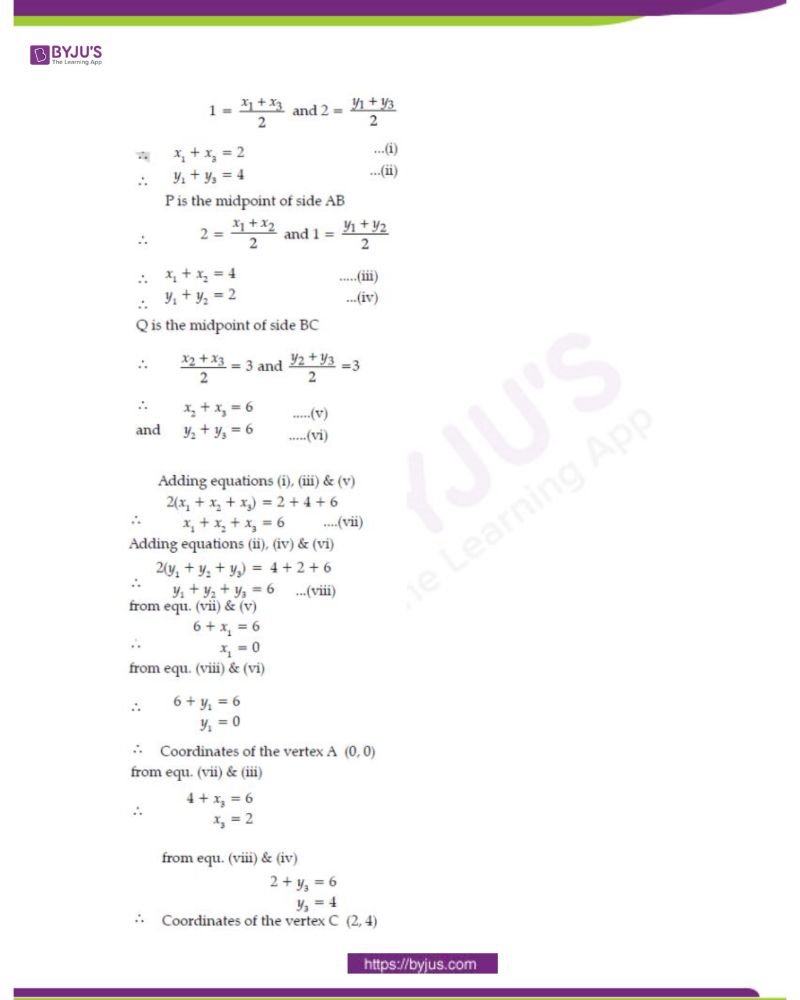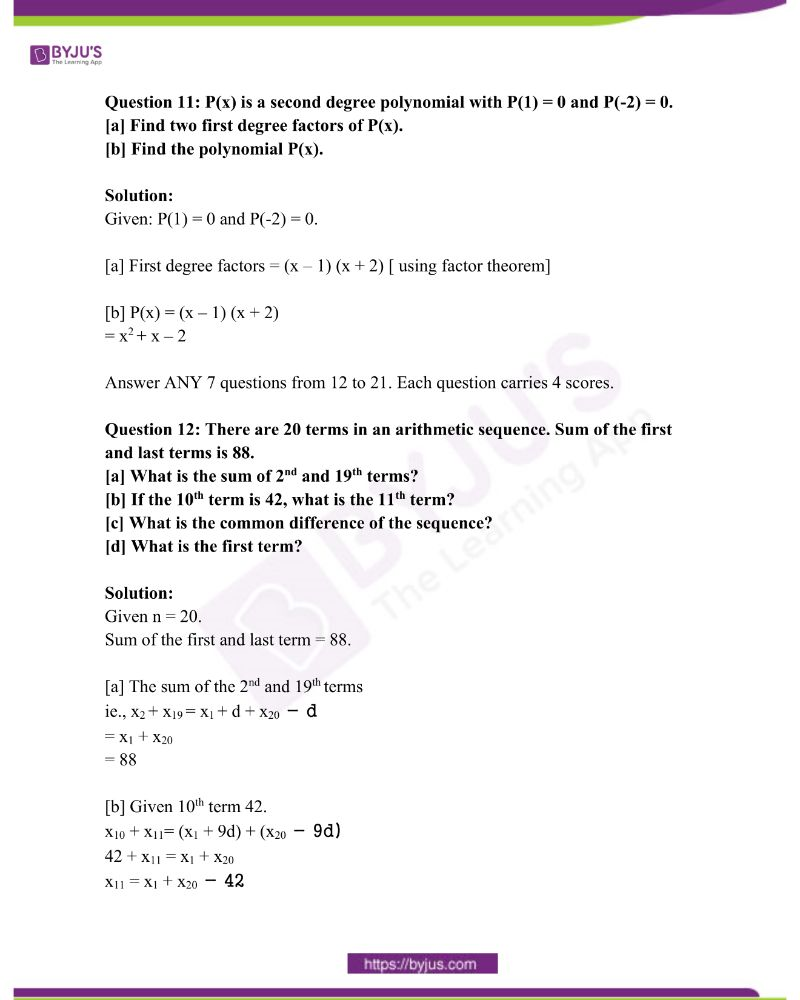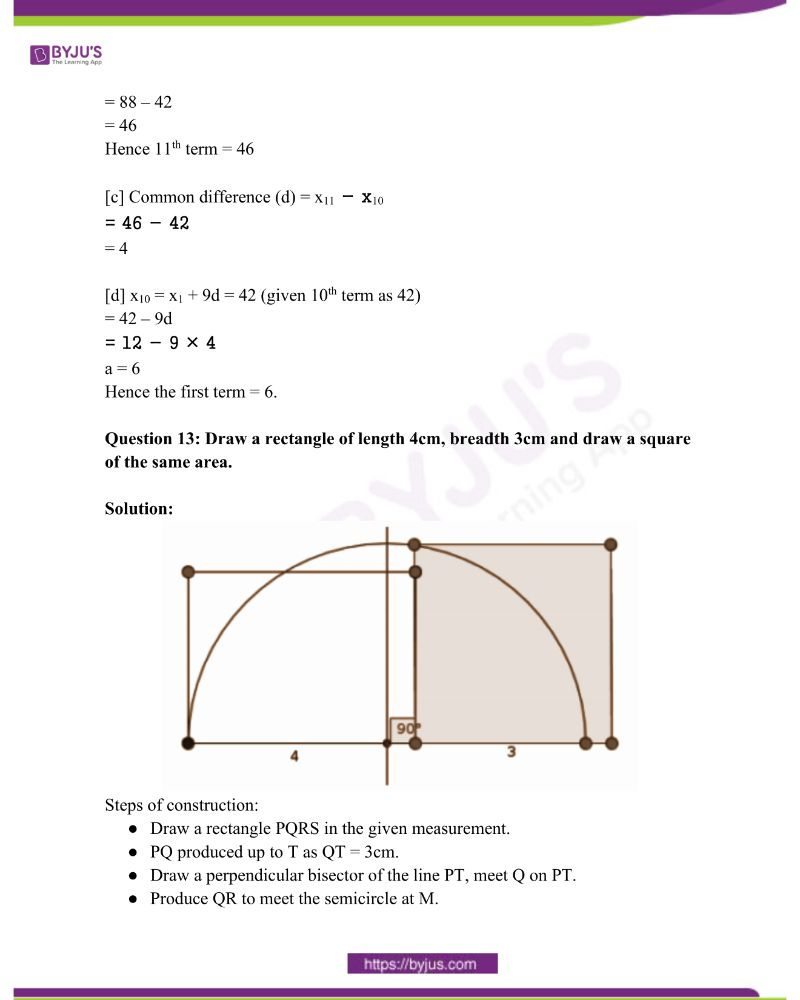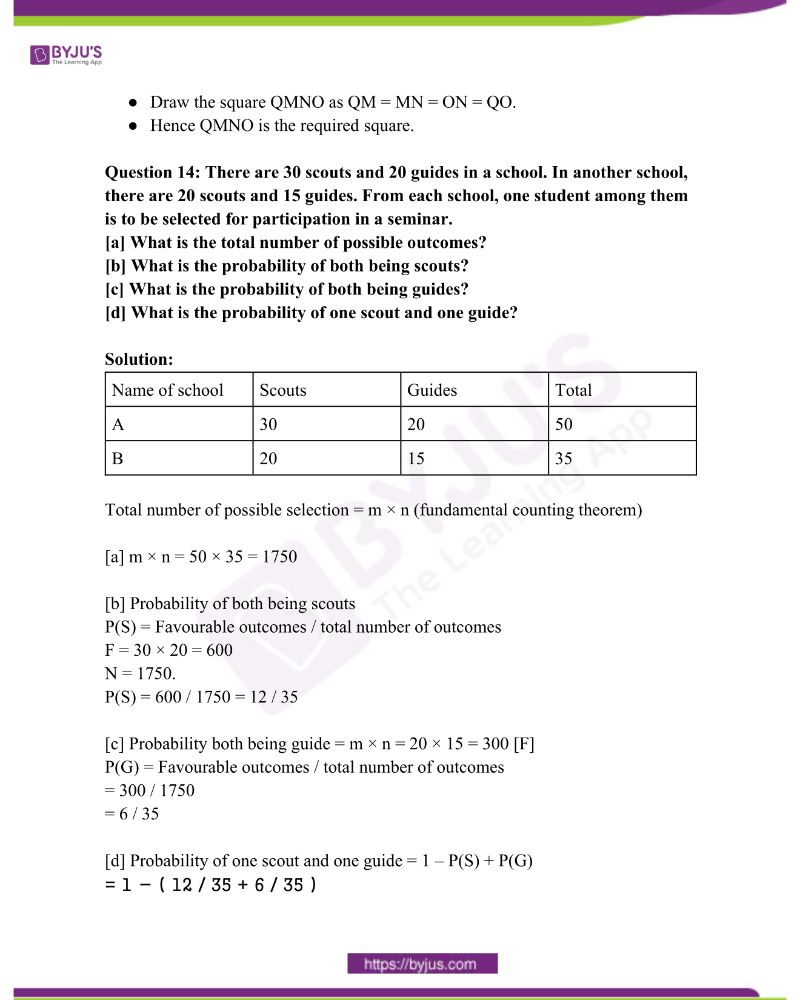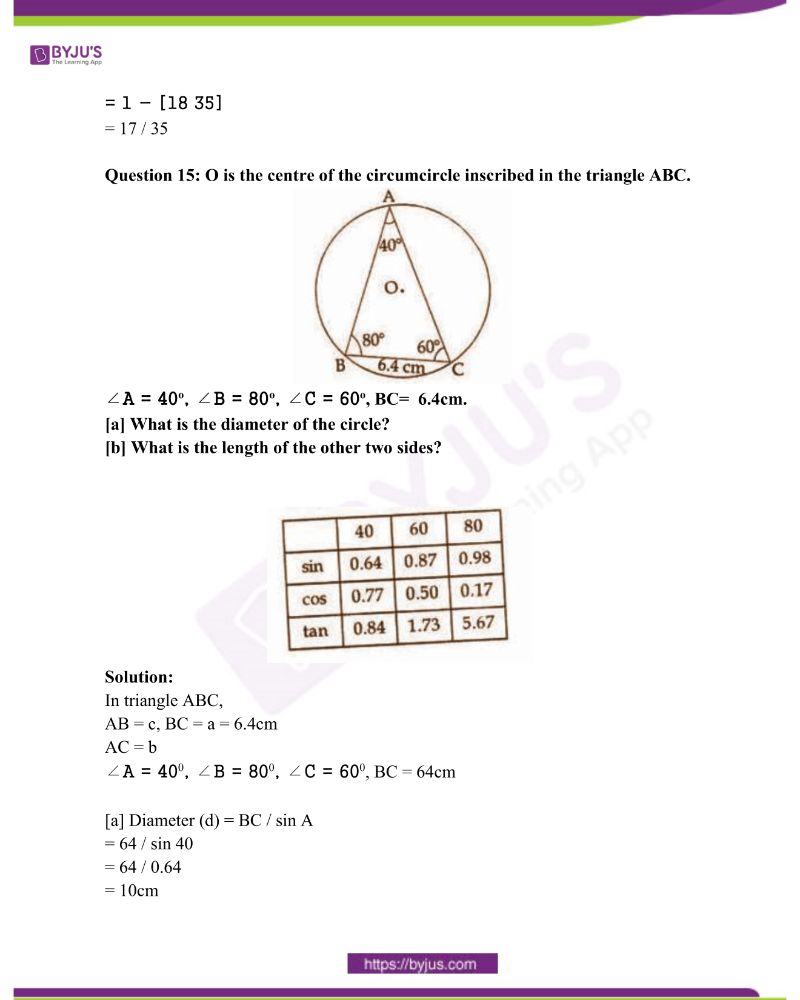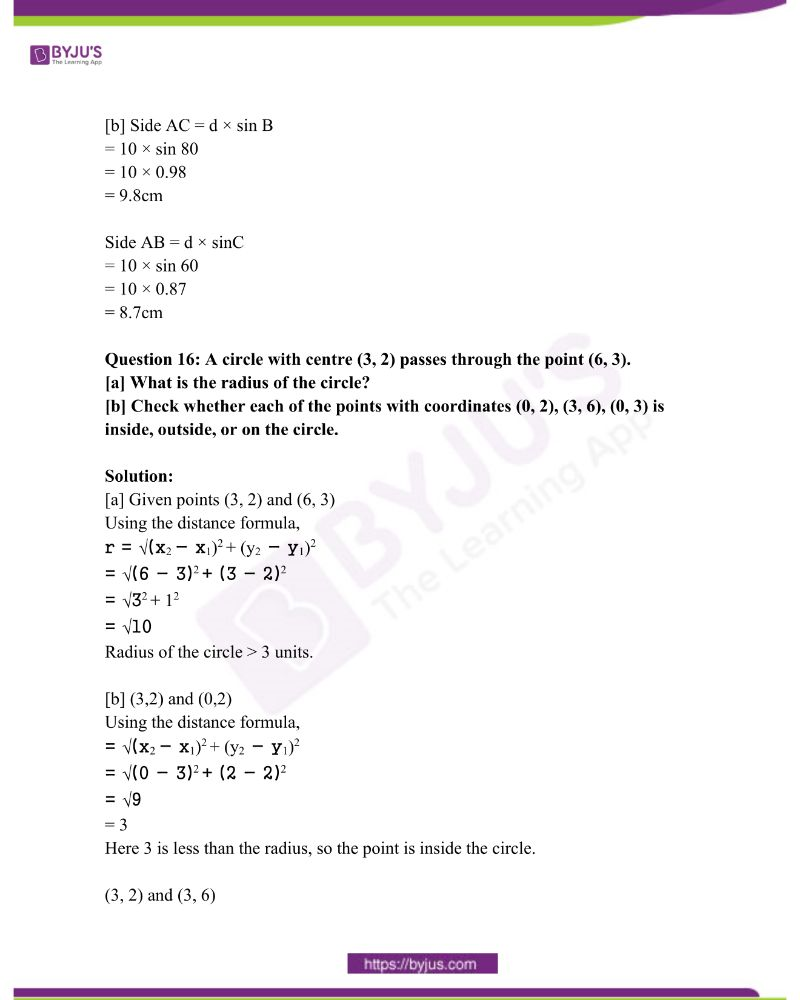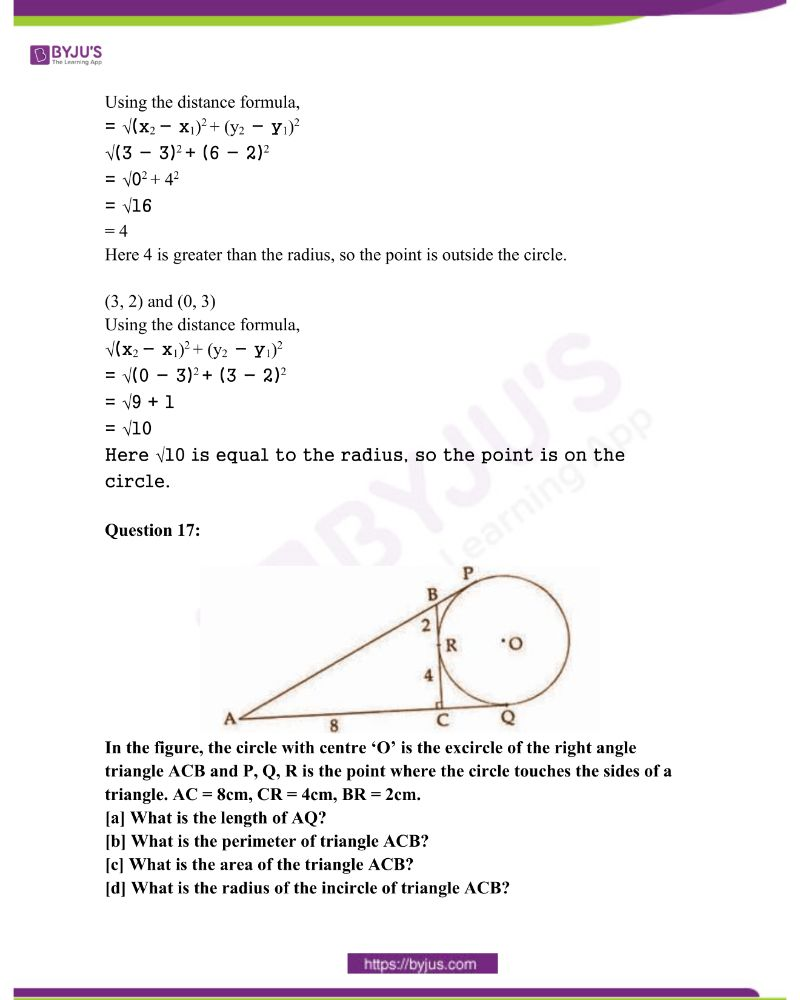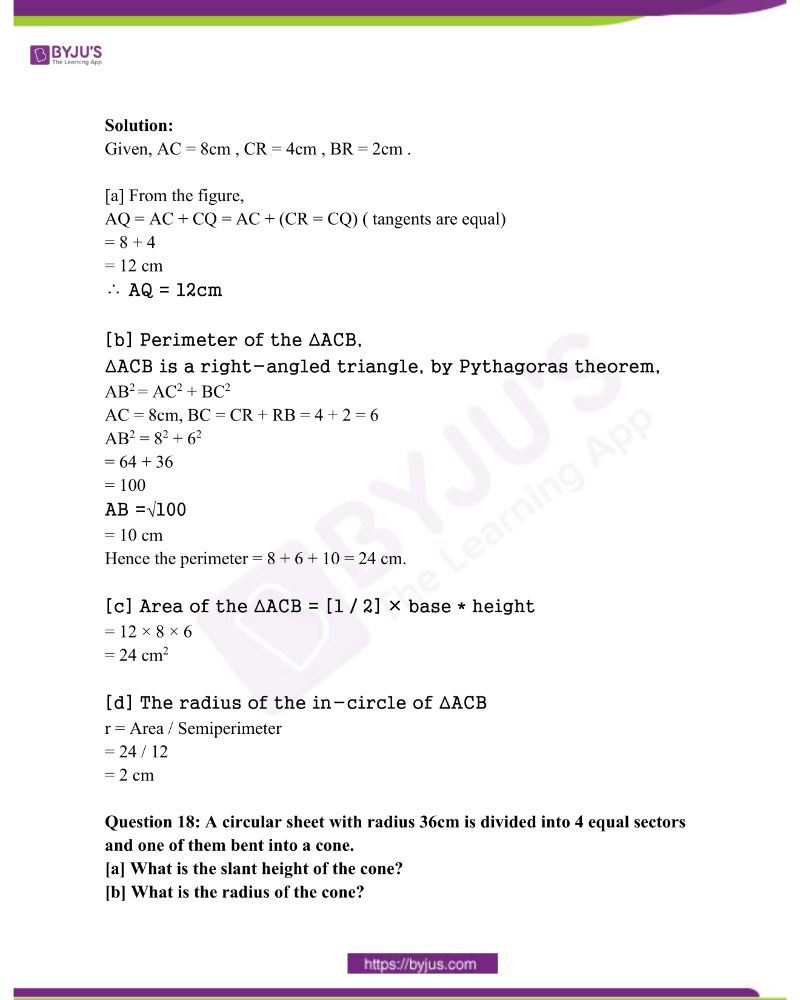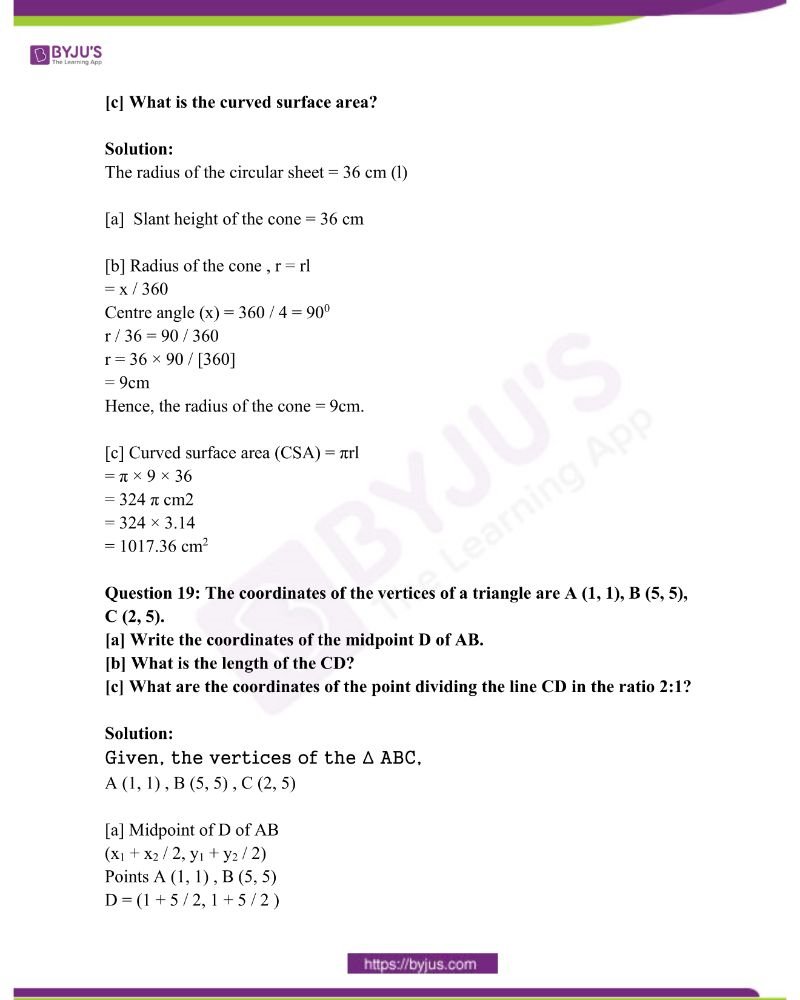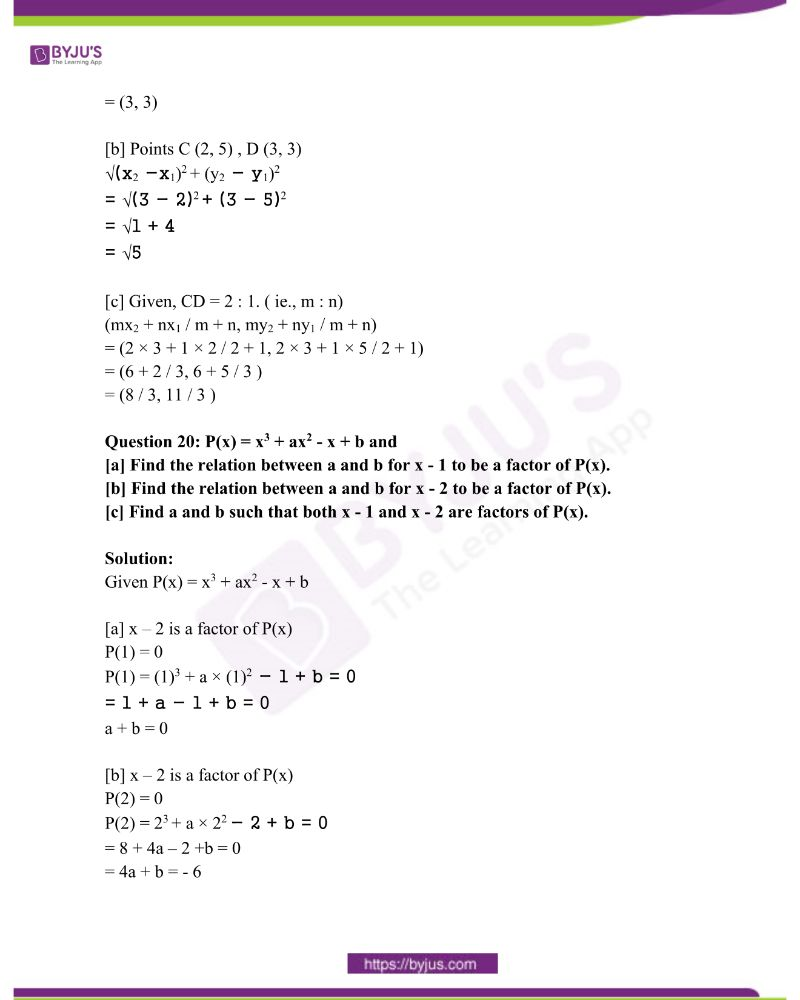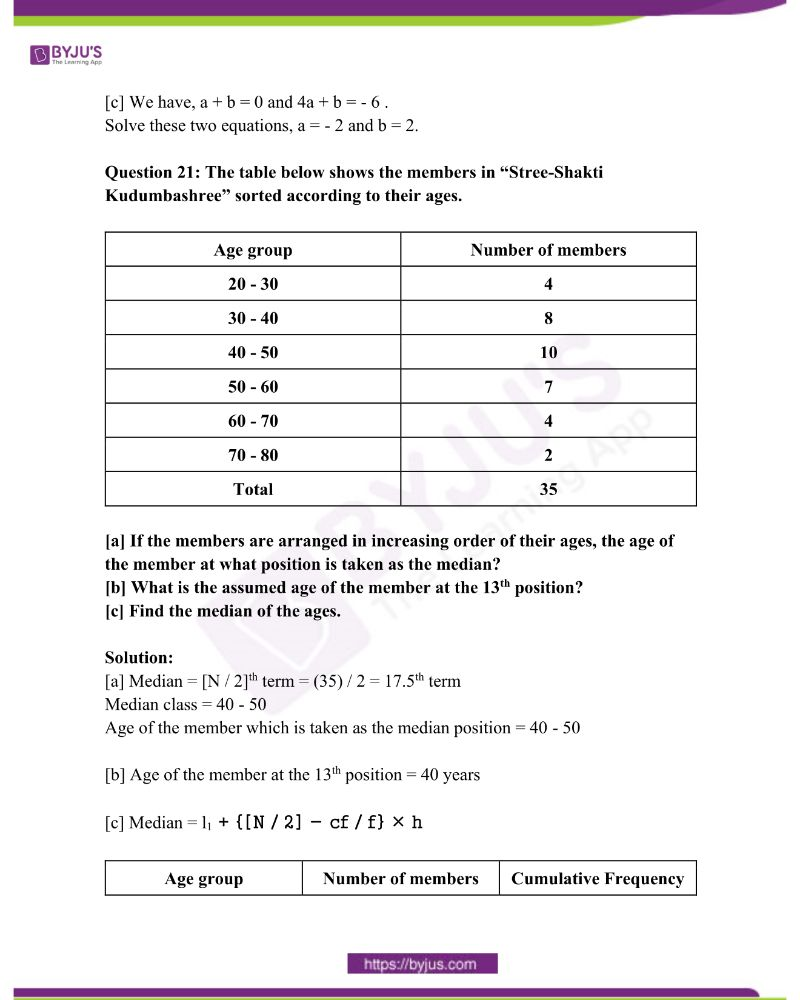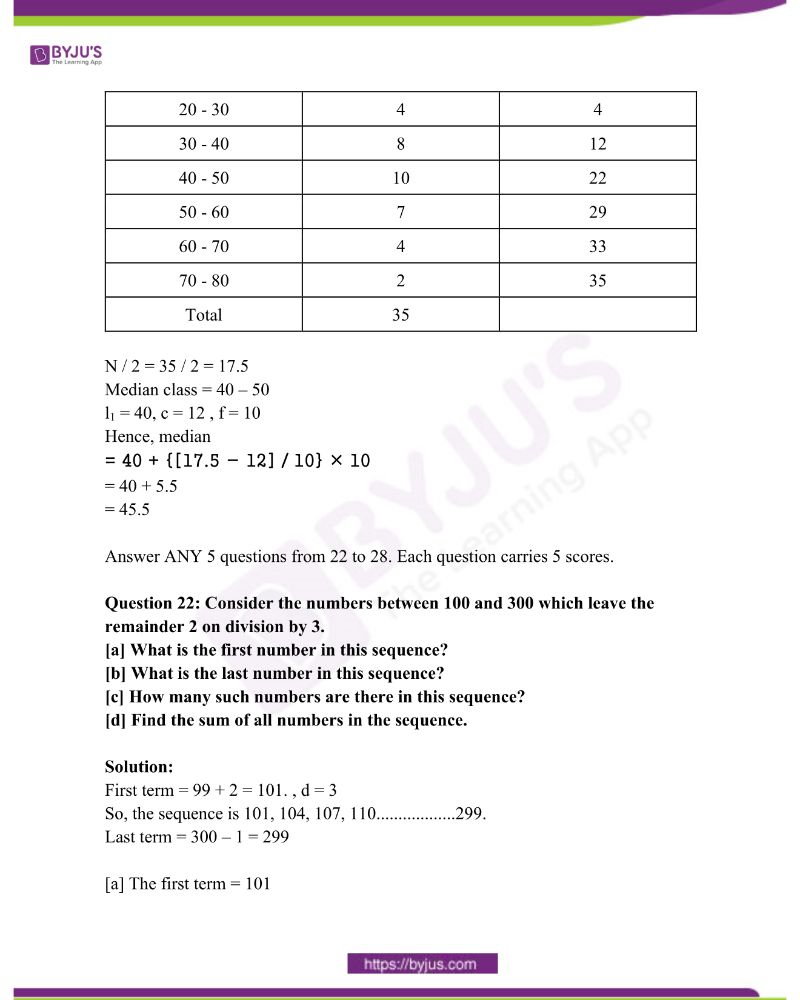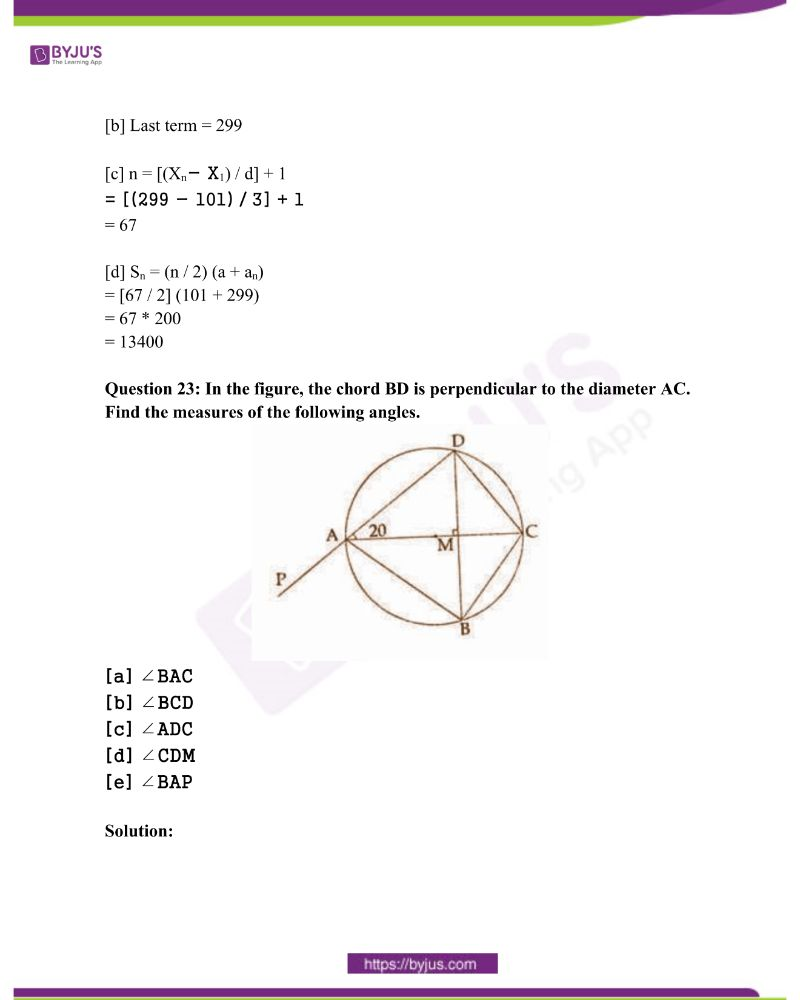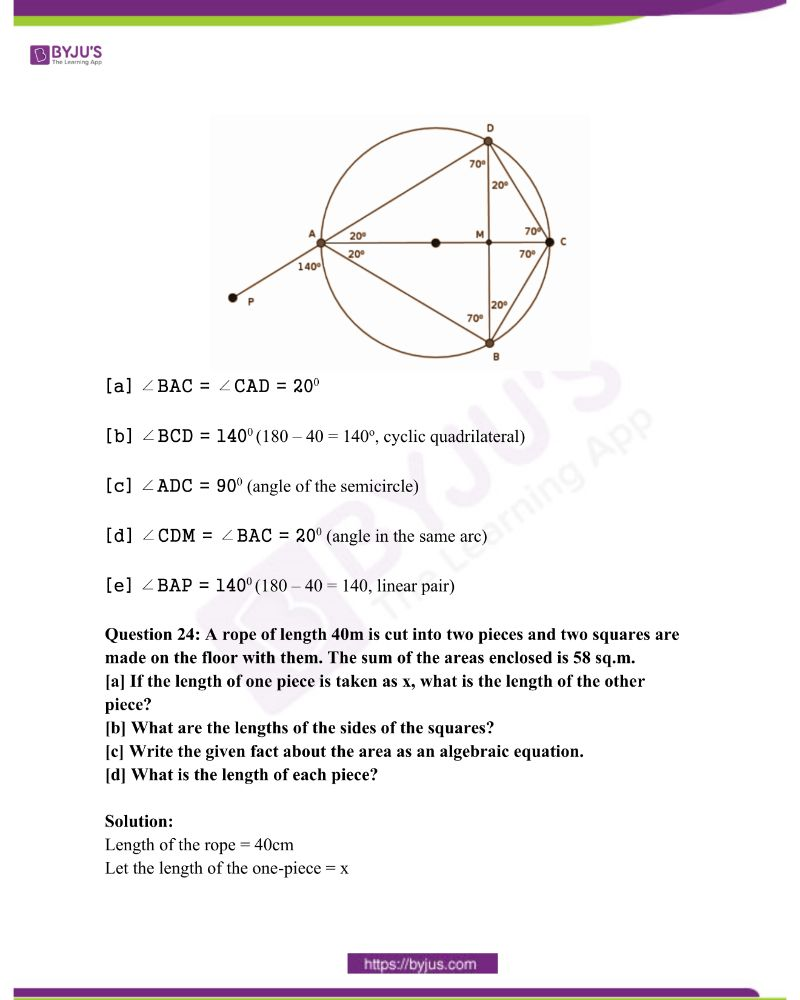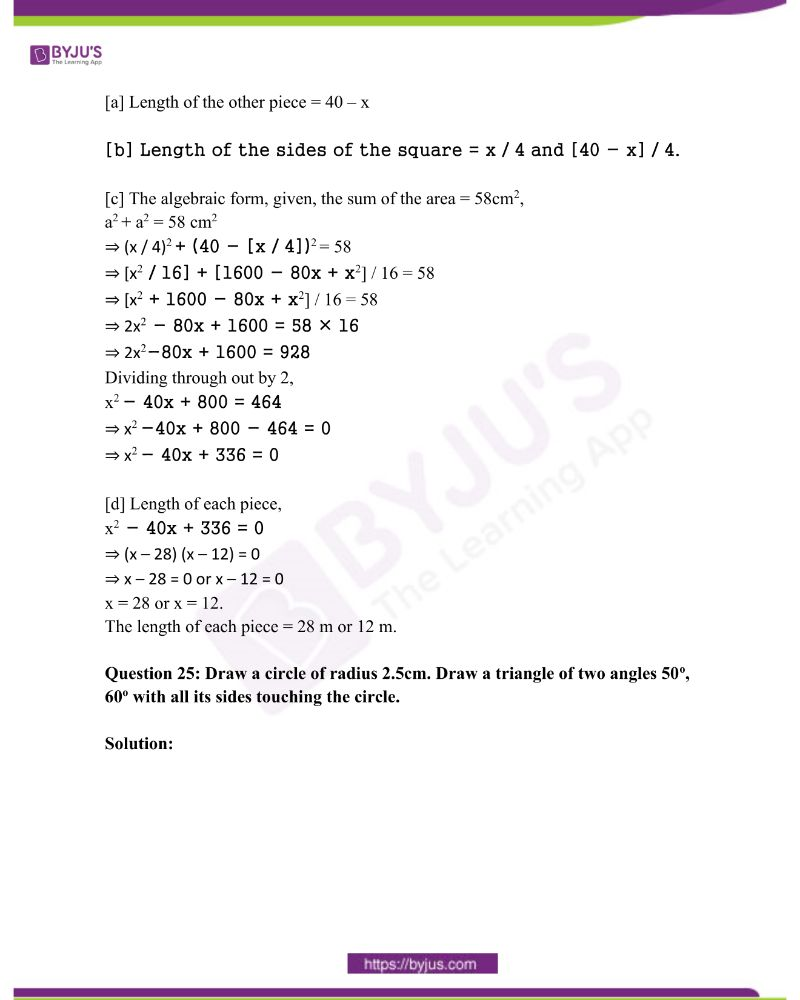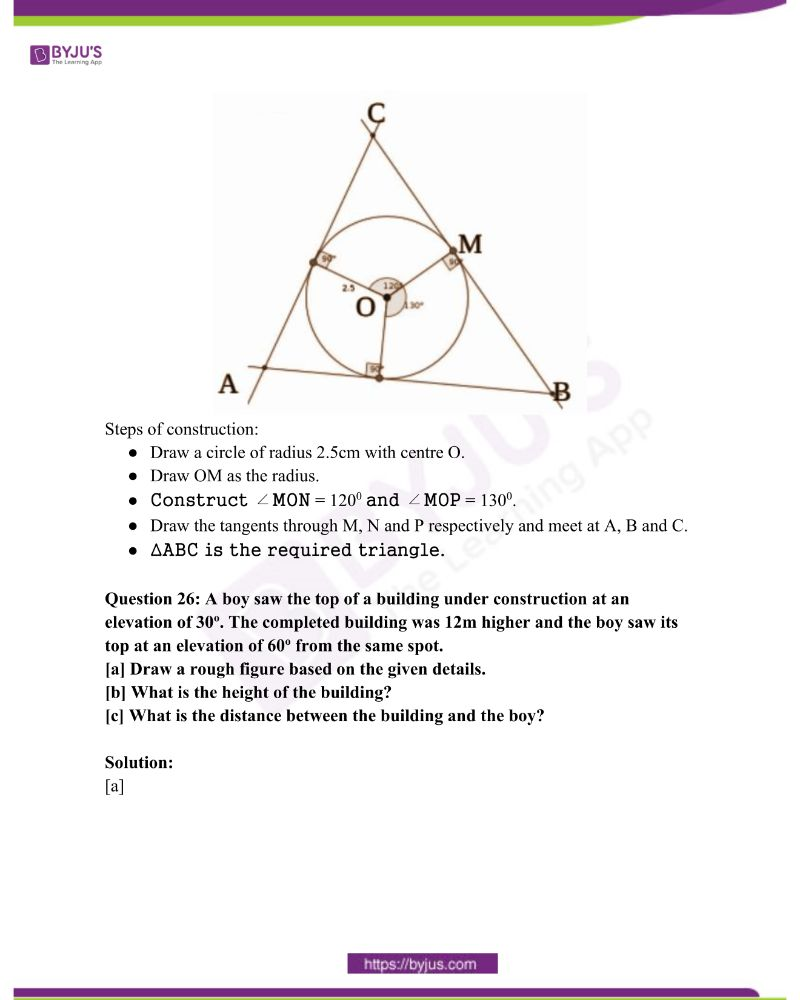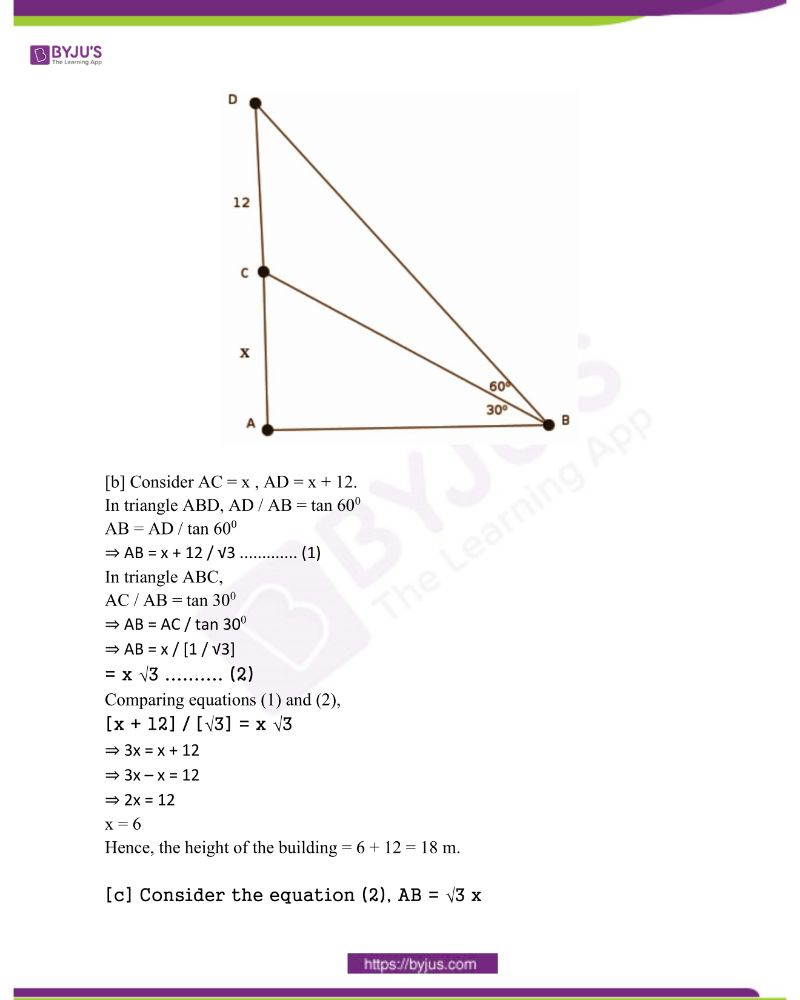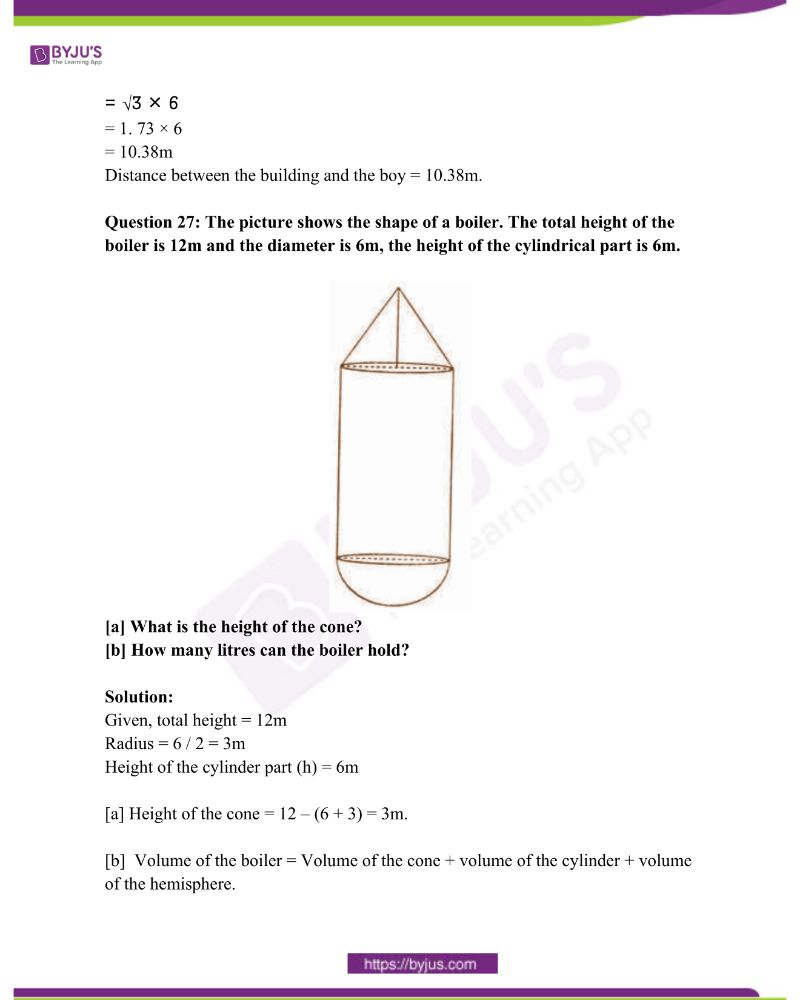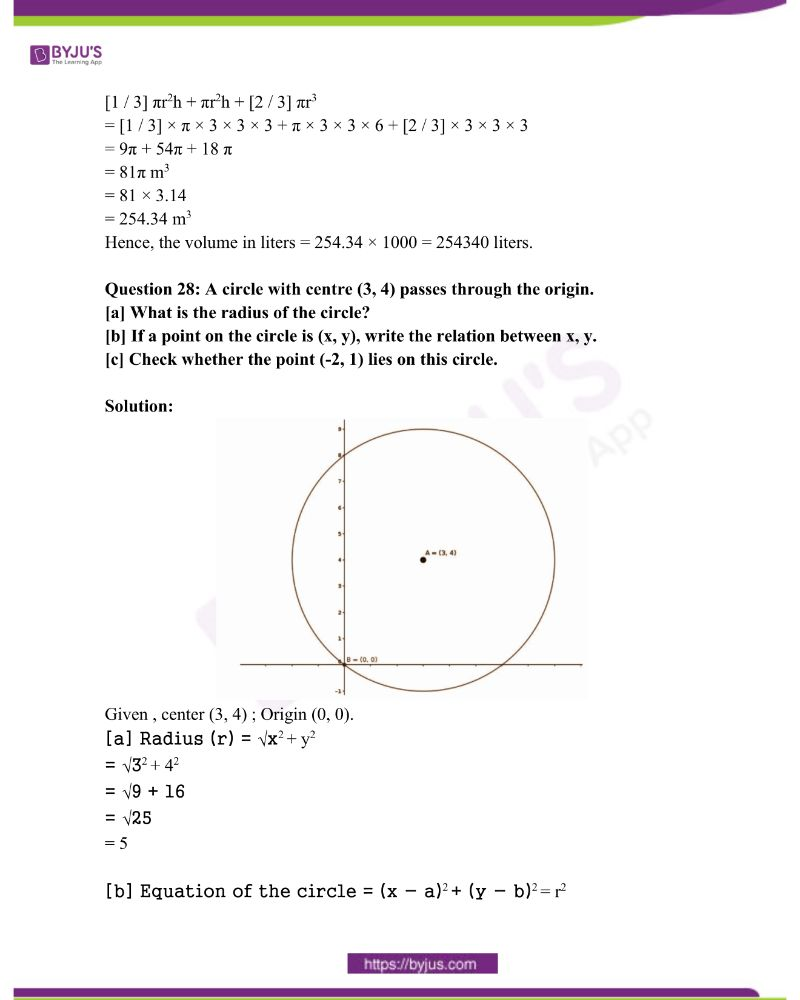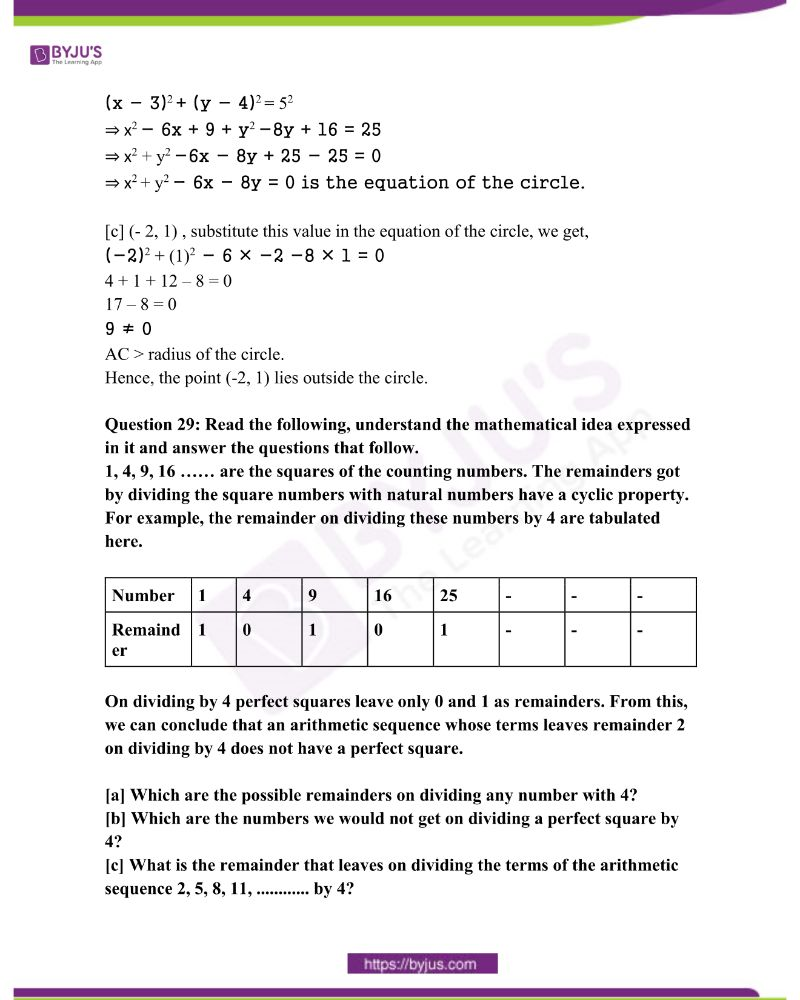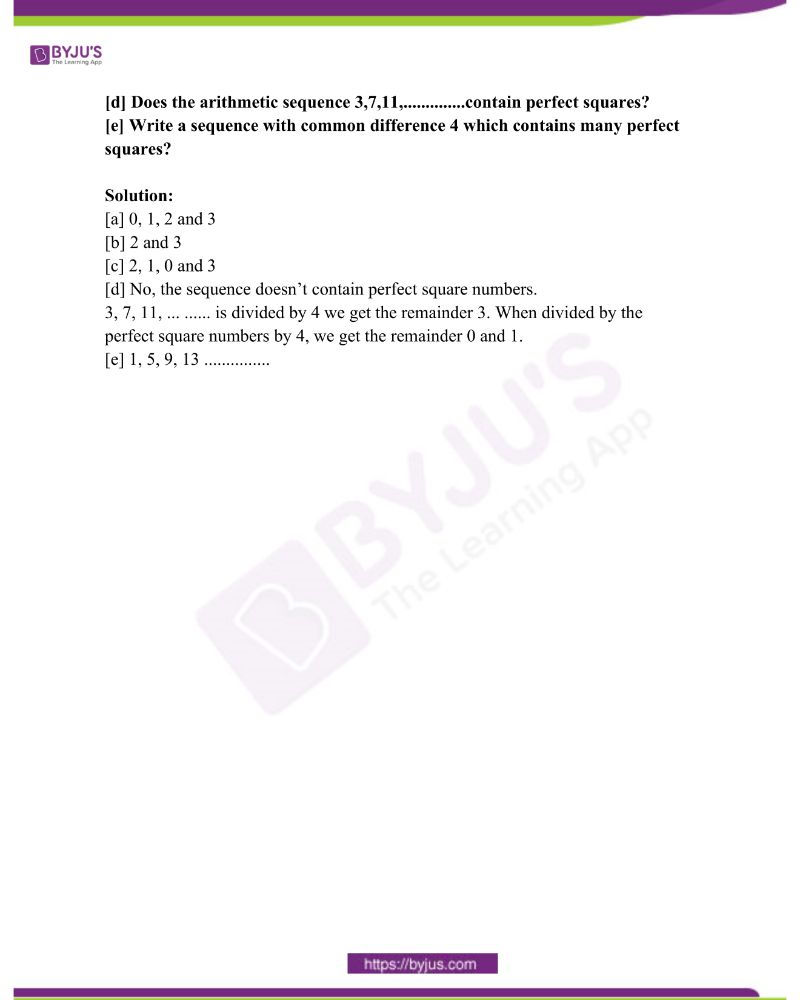Answer ANY 3 questions from 1 to 4. Each question carries 2 scores.

Question 1: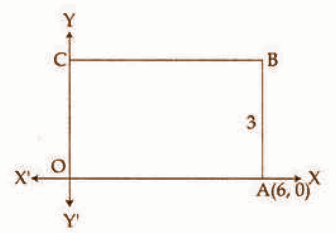In the figure, OABC is a rectangle and its breadth is 3. Write the coordinates of the vertices B and C.

Solution:

Given OABC is a rectangle.

A (6, 0)

From the figure AB = 3 cm (breadth)

Hence, B = (6 , 3) and C = (0 , 3) [OC = 3cm breadth]

Question 2: The letters of the word MALAYALAM are written in paper slips and put into a box. A child is asked to take one slip from the box without looking.

[a] What is the probability of getting the letter A?

[b] What is the probability of not getting the letter A?

Solution:

MALAYALAM

Total letters = 9

n(S) = 9

Number of times A occurs = 4

n(E) = 4

[a] Probability of getting letter A = Favourable events / Total number of outcomes

= n(E) / n(S)

= 4 / 9

[b] Probability of not getting A = 1 – P(E) = 1 – [4 / 9] = 5 / 9

Question 3: The algebraic form of an arithmetic sequence is 5n + 3.

[a] What is the first term of the sequence?

[b] What will be the remainder if the terms of the sequence are divided by 5?

Solution:

an = 5n + 3

[a] To obtain the first term of the sequence, put n = 1,

a1 = 5 × 1 + 3 = 8

[b] d = 5 (coefficient of n be a common difference )

The remainder obtained when divided by 5 = 3 [8 / 5 = 3, 13 / 5 = 3, 18 / 5 = 3,.etc.]

Question 4: The weights of 11 children in a school cricket club are 35, 39, 32, 36, 40, 30, 34, 37, 38, 33, 31 (kgs). Find the median weight.

Solution:

Weight of 11 children in ascending order (in kgs)

30, 31, 32, 33, 34, 35, 36, 37, 38, 39, 40

Here n = 11, which is odd.

So, median = {[n + 1]th / 2} term = [11 + 1]th / 2 = 6th term

The median weight of children = 35kg.

Answer ANY 5 questions from 5 to 11. Each question carries 3 scores.

Question 5: In the figure, O is the centre of the circle and A, B, C, D, E are the points on it.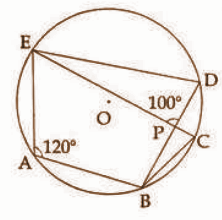∠EAB = 120o, ∠EPD = 100o. Write the measures of ∠EDB, ∠ECB and ∠DBC.

Solution:

∠EDB + ∠EAB = 180o

120o + ∠EDB = 180o

∠EDB = 60o

∠EAB + ∠ECB = 180o

120o + ∠ECB = 180o

∠ECB = 60o

∠BPE = ∠DPE [vertically opposite angles]

∠BPE = 100o

In triangle BPC,

∠BPC + ∠BCP + ∠PBC = 180o

100o + 60o + ∠PBC = 180o

∠PBC = 180o – 160o

∠PBC = 20o

∠DBC = 20o [same arc angles]

Question 6: The algebraic form for the sum of first n terms of an arithmetic sequence is 2n2 + 8n. How many consecutive terms of the sequence, starting from the first, are to be added to get 330?

Solution:

Sn = 2n2 + 8n

330 = 2n2 + 8n

2n2 + 8n – 330 = 0

n2 – 4n – 165 = 0

n(n + 15) – 11 (n + 15) = 0

(n + 15) (n – 11) = 0

n = 11, -15

Since the number of terms cannot be negative, n = 11.

Question 7: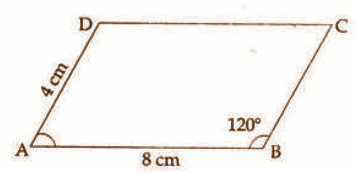ABCD is a parallelogram. AB = 8cm, AD = 4cm, ∠B = 120o

[a] What is ∠A?

[b] What is the perpendicular distance from D to AB?

[c] What is the area of ABCD?

Solution:

Given ABCD is a parallelogram.

AB = 8 cm , AD = 4cm , ∠B = 1200

[a] ∠B = ∠D = 1200 ( opposite angles equal)

∠ A + ∠C = 360 – (120 + 120)

= 360 – 240

= 120

∴∠ A = ∠C = 120 / 2 = 60o

∴∠ A = 600

[b] Draw DE as perpendicular from D to AB.

In triangle APD, ∠P = 90o,

DP / AD = sin A

DP / 4 = sin 60o

DP / 4 = √3 / 2

DP = √3 / 2 * 4

= 2√3 cm

[c] The area of parallelogram ABCD = base * height

= 8 × 2 √3

= 16√3 cm2

Question 8: Draw a circle of radius 3cm. Mark a point 7cm away from the centre. Draw the tangents to the circle from this point.

Solution: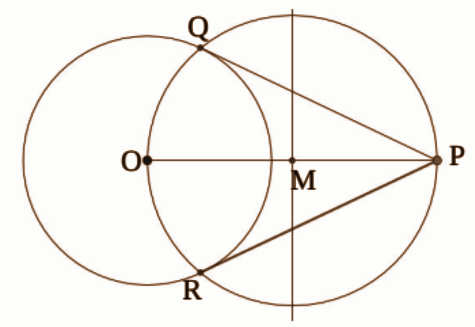Steps of construction:

• Draw a circle of 3cm radius and O as its centre.
• From the centre, mark OP = 7cm.
• Draw a perpendicular bisector of the line OP such that it meets at M.
• Draw a circle with radius OM and cut the circle at Q and R.
• Joint PR and PQ.
• Hence, PR and PQ are tangents.

Question 9: The perimeter of the base of a square pyramid is 96cm and its height is 16cm.

[a] What is the length of a base edge?

[b] What is the slant height?

[c] Find the lateral surface area.

Solution:

The perimeter of the base of a square pyramid = 96cm

Height = 16cm

[a] 4* base edge = 96

4a = 96

a = 96 / 4

= 24cm

∴ Base edge = 24cm.

[b] Slant height (l) = l2 = √(base / 2)2 + (height)2

= √(24 / 2)2 + 162

= √122 + 162

= √144 + 256

= √400

= 20 cm

[c] Lateral surface area (LSA) = (1 / 2) * perimeter * slant height

= (1 / 2) × 96 × 20

= 960 cm2

Question 10: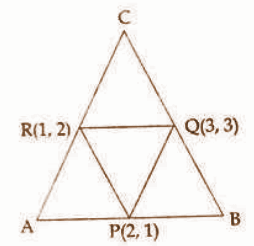P, Q, R are the midpoints of the sides of triangle ABC.

[a] What type of quadrilateral is PQCR?

[b] Write the coordinates of the vertices A and C.

Solution:

[a] (a) Q & R are midpoints of sides BC and AC, respectively

∴ QR || AB

Similarly PQ || AC

∴ PQCR is a parallelogram.

[b] Let the vertex of A, B and C be (x1, y1), (x2, y2) and (x3, y3) respectively R is the midpoint of AC.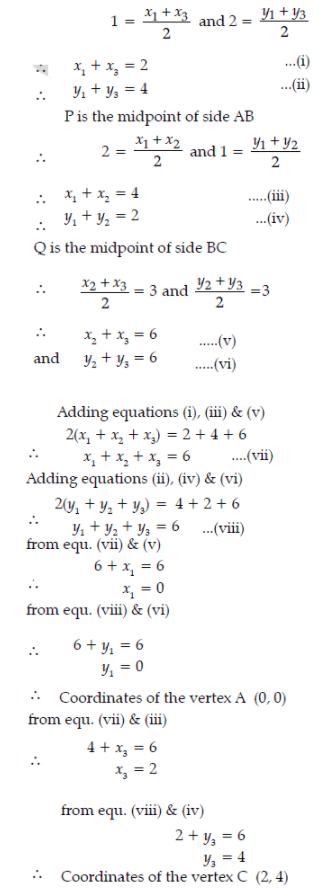Question 11: P(x) is a second degree polynomial with P(1) = 0 and P(-2) = 0.

[a] Find two first degree factors of P(x).

[b] Find the polynomial P(x).

Solution:

Given: P(1) = 0 and P(-2) = 0.

[a] First degree factors = (x – 1) (x + 2) [ using factor theorem] [b] P(x) = (x – 1) (x + 2)

= x2 + x – 2

Answer ANY 7 questions from 12 to 21. Each question carries 4 scores.

Question 12: There are 20 terms in an arithmetic sequence. Sum of the first and last terms is 88.

[a] What is the sum of 2nd and 19th terms?

[b] If the 10th term is 42, what is the 11th term?

[c] What is the common difference of the sequence?

[d] What is the first term?

Solution:

Given n = 20.

Sum of the first and last term = 88.

[a] The sum of the 2nd and 19th terms

ie., x2 + x19 = x1 + d + x20 − d

= x1 + x20

= 88

[b] Given 10th term 42.

x10 + x11= (x1 + 9d) + (x20 − 9d)

42 + x11 = x1 + x20

x11 = x1 + x20 − 42

= 88 – 42

= 46

Hence 11th term = 46

[c] Common difference (d) = x11 − x10

= 46 − 42

= 4

[d] x10 = x1 + 9d = 42 (given 10th term as 42)

= 42 – 9d

= 12 − 9 × 4

a = 6

Hence the first term = 6.

Question 13: Draw a rectangle of length 4cm, breadth 3cm and draw a square of the same area.

Solution: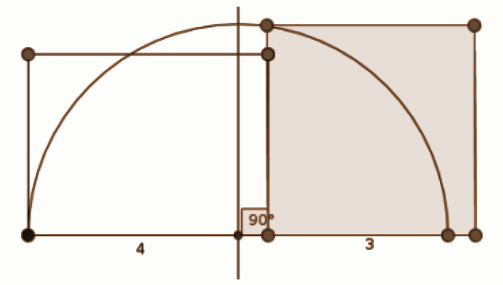Steps of construction:

• Draw a rectangle PQRS in the given measurement.
• PQ produced up to T as QT = 3cm.
• Draw a perpendicular bisector of the line PT, meet Q on PT.
• Produce QR to meet the semicircle at M.
• Draw the square QMNO as QM = MN = ON = QO.
• Hence QMNO is the required square.

Question 14: There are 30 scouts and 20 guides in a school. In another school, there are 20 scouts and 15 guides. From each school, one student among them is to be selected for participation in a seminar.

[a] What is the total number of possible outcomes?

[b] What is the probability of both being scouts?

[c] What is the probability of both being guides?

[d] What is the probability of one scout and one guide?

Solution:

 Name of school Scouts Guides Total A 30 20 50 B 20 15 35

Total number of possible selection = m × n (fundamental counting theorem)

[a] m × n = 50 × 35 = 1750

[b] Probability of both being scouts

P(S) = Favourable outcomes / total number of outcomes

F = 30 × 20 = 600

N = 1750.

P(S) = 600 / 1750 = 12 / 35

[c] Probability both being guide = m × n = 20 × 15 = 300 [F]

P(G) = Favourable outcomes / total number of outcomes

= 300 / 1750

= 6 / 35

[d] Probability of one scout and one guide = 1 – P(S) + P(G)

= 1 − ( 12 / 35 + 6 / 35 )

= 1 − [18 35]

= 17 / 35

Question 15: O is the centre of the circumcircle inscribed in the triangle ABC.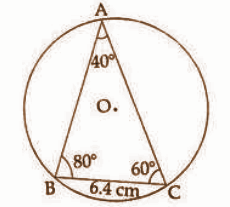∠A = 40o, ∠B = 80o, ∠C = 60o, BC= 6.4cm.

[a] What is the diameter of the circle?

[b] What is the length of the other two sides?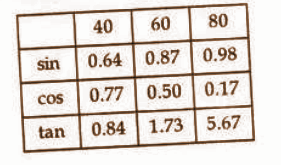Solution:

In triangle ABC,

AB = c, BC = a = 6.4cm

AC = b

∠A = 400, ∠B = 800, ∠C = 600, BC = 64cm

[a] Diameter (d) = BC / sin A

= 64 / sin 40

= 64 / 0.64

= 10cm

[b] Side AC = d × sin B

= 10 × sin 80

= 10 × 0.98

= 9.8cm

Side AB = d × sinC

= 10 × sin 60

= 10 × 0.87

= 8.7cm

Question 16: A circle with centre (3, 2) passes through the point (6, 3).

[a] What is the radius of the circle?

[b] Check whether each of the points with coordinates (0, 2), (3, 6), (0, 3) is inside, outside, or on the circle.

Solution:

[a] Given points (3, 2) and (6, 3)

Using the distance formula,

r = √(x2 − x1)2 + (y2 − y1)2

= √(6 − 3)2 + (3 − 2)2

= √32 + 12

= √10

Radius of the circle > 3 units.

[b] (3,2) and (0,2)

Using the distance formula,

= √(x2 − x1)2 + (y2 − y1)2

= √(0 − 3)2 + (2 − 2)2

= √9

= 3

Here 3 is less than the radius, so the point is inside the circle.

(3, 2) and (3, 6)

Using the distance formula,

= √(x2 − x1)2 + (y2 − y1)2

√(3 − 3)2 + (6 − 2)2

= √02 + 42

= √16

= 4

Here 4 is greater than the radius, so the point is outside the circle.

(3, 2) and (0, 3)

Using the distance formula,

√(x2 − x1)2 + (y2 − y1)2

= √(0 − 3)2 + (3 − 2)2

= √9 + 1

= √10

Here √10 is equal to the radius, so the point is on the circle.

Question 17: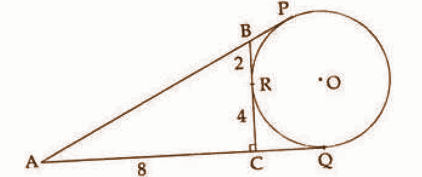In the figure, the circle with centre ‘O’ is the excircle of the right angle triangle ACB and P, Q, R is the point where the circle touches the sides of a triangle. AC = 8cm, CR = 4cm, BR = 2cm.

[a] What is the length of AQ?

[b] What is the perimeter of triangle ACB?

[c] What is the area of the triangle ACB?

[d] What is the radius of the incircle of triangle ACB?

Solution:

Given, AC = 8cm , CR = 4cm , BR = 2cm .

[a] From the figure,

AQ = AC + CQ = AC + (CR = CQ) ( tangents are equal)

= 8 + 4

= 12 cm

∴ AQ = 12cm

[b] Perimeter of the ∆ACB,

∆ACB is a right-angled triangle, by Pythagoras theorem,

AB2 = AC2 + BC2

AC = 8cm, BC = CR + RB = 4 + 2 = 6

AB2 = 82 + 62

= 64 + 36

= 100

AB =√100

= 10 cm

Hence the perimeter = 8 + 6 + 10 = 24 cm.

[c] Area of the ∆ACB = [1 / 2] × base * height

= 12 × 8 × 6

= 24 cm2

[d] The radius of the in-circle of ∆ACB

r = Area / Semiperimeter

= 24 / 12

= 2 cm

Question 18: A circular sheet with radius 36cm is divided into 4 equal sectors and one of them bent into a cone.

[a] What is the slant height of the cone?

[b] What is the radius of the cone?

[c] What is the curved surface area?

Solution:

The radius of the circular sheet = 36 cm (l)

[a] Slant height of the cone = 36 cm

[b] Radius of the cone , r = rl

= x / 360

Centre angle (x) = 360 / 4 = 900

r / 36 = 90 / 360

r = 36 × 90 / 

= 9cm

Hence, the radius of the cone = 9cm.

[c] Curved surface area (CSA) = πrl

= π × 9 × 36

= 324 π cm2

= 324 × 3.14

= 1017.36 cm2

Question 19: The coordinates of the vertices of a triangle are A (1, 1), B (5, 5), C (2, 5).

[a] Write the coordinates of the midpoint D of AB.

[b] What is the length of the CD?

[c] What are the coordinates of the point dividing the line CD in the ratio 2:1?

Solution:

Given, the vertices of the ∆ ABC,

A (1, 1) , B (5, 5) , C (2, 5)

[a] Midpoint of D of AB

(x1 + x2 / 2, y1 + y2 / 2)

Points A (1, 1) , B (5, 5)

D = (1 + 5 / 2, 1 + 5 / 2 )

= (3, 3)

[b] Points C (2, 5) , D (3, 3)

√(x2 −x1)2 + (y2 − y1)2

= √(3 − 2)2 + (3 − 5)2

= √1 + 4

= √5

[c] Given, CD = 2 : 1. ( ie., m : n)

(mx2 + nx1 / m + n, my2 + ny1 / m + n)

= (2 × 3 + 1 × 2 / 2 + 1, 2 × 3 + 1 × 5 / 2 + 1)

= (6 + 2 / 3, 6 + 5 / 3 )

= (8 / 3, 11 / 3 )

Question 20: P(x) = x3 + ax2 – x + b and

[a] Find the relation between a and b for x – 1 to be a factor of P(x).

[b] Find the relation between a and b for x – 2 to be a factor of P(x).

[c] Find a and b such that both x – 1 and x – 2 are factors of P(x).

Solution:

Given P(x) = x3 + ax2 – x + b

[a] x – 2 is a factor of P(x)

P(1) = 0

P(1) = (1)3 + a × (1)2 − 1 + b = 0

= 1 + a − 1 + b = 0

a + b = 0

[b] x – 2 is a factor of P(x)

P(2) = 0

P(2) = 23 + a × 22 − 2 + b = 0

= 8 + 4a – 2 +b = 0

= 4a + b = – 6

[c] We have, a + b = 0 and 4a + b = – 6 .

Solve these two equations, a = – 2 and b = 2.

Question 21: The table below shows the members in “Stree-Shakti Kudumbashree” sorted according to their ages.

 Age group Number of members 20 – 30 4 30 – 40 8 40 – 50 10 50 – 60 7 60 – 70 4 70 – 80 2 Total 35

[a] If the members are arranged in increasing order of their ages, the age of the member at what position is taken as the median?

[b] What is the assumed age of the member at the 13th position?

[c] Find the median of the ages.

Solution:

[a] Median = [N / 2]th term = (35) / 2 = 17.5th term

Median class = 40 – 50

Age of the member which is taken as the median position = 40 – 50

[b] Age of the member at the 13th position = 40 years

[c] Median = l1 + {[N / 2] − cf / f} × h

 Age group Number of members Cumulative Frequency 20 – 30 4 4 30 – 40 8 12 40 – 50 10 22 50 – 60 7 29 60 – 70 4 33 70 – 80 2 35 Total 35

N / 2 = 35 / 2 = 17.5

Median class = 40 – 50

l1 = 40, c = 12 , f = 10

Hence, median

= 40 + {[17.5 − 12] / 10} × 10

= 40 + 5.5

= 45.5

Answer ANY 5 questions from 22 to 28. Each question carries 5 scores.

Question 22: Consider the numbers between 100 and 300 which leave the remainder 2 on division by 3.

[a] What is the first number in this sequence?

[b] What is the last number in this sequence?

[c] How many such numbers are there in this sequence?

[d] Find the sum of all numbers in the sequence.

Solution:

First term = 99 + 2 = 101. , d = 3

So, the sequence is 101, 104, 107, 110………………299.

Last term = 300 – 1 = 299

[a] The first term = 101

[b] Last term = 299

[c] n = [(Xn− X1) / d] + 1

= [(299 − 101) / 3] + 1

= 67

[d] Sn = (n / 2) (a + an)

= [67 / 2] (101 + 299)

= 67 * 200

= 13400

Question 23: In the figure, the chord BD is perpendicular to the diameter AC. Find the measures of the following angles.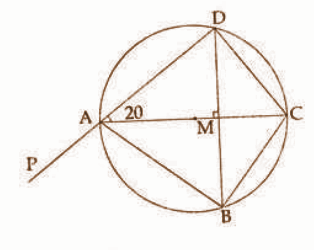[a] ∠BAC

[b] ∠BCD

[d] ∠CDM

[e] ∠BAP

Solution: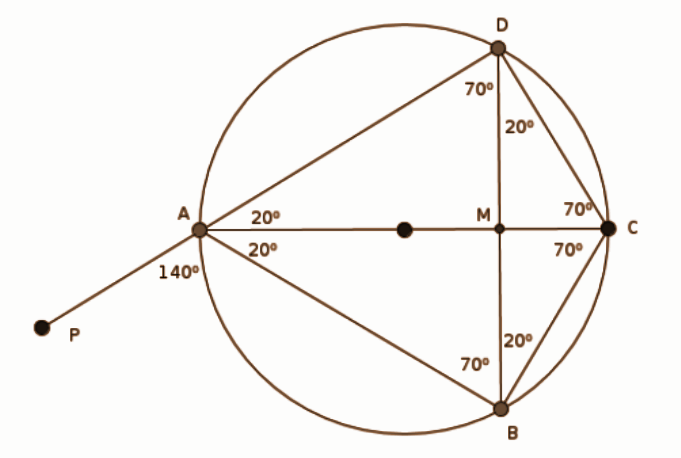[a] ∠BAC = ∠CAD = 200

[b] ∠BCD = 1400 (180 – 40 = 140o, cyclic quadrilateral)

[c] ∠ADC = 900 (angle of the semicircle)

[d] ∠CDM = ∠BAC = 200 (angle in the same arc)

[e] ∠BAP = 1400 (180 – 40 = 140, linear pair)

Question 24: A rope of length 40m is cut into two pieces and two squares are made on the floor with them. The sum of the areas enclosed is 58 sq.m.

[a] If the length of one piece is taken as x, what is the length of the other piece?

[b] What are the lengths of the sides of the squares?

[c] Write the given fact about the area as an algebraic equation.

[d] What is the length of each piece?

Solution:

Length of the rope = 40cm

Let the length of the one-piece = x

[a] Length of the other piece = 40 – x

[b] Length of the sides of the square = x / 4 and [40 − x] / 4.

[c] The algebraic form, given, the sum of the area = 58cm2,

a2 + a2 = 58 cm2

⇒ (x / 4)2 + (40 − [x / 4])2 = 58

⇒ [x2 / 16] + [1600 − 80x + x2] / 16 = 58

⇒ [x2 + 1600 − 80x + x2] / 16 = 58

⇒ 2x2 − 80x + 1600 = 58 × 16

⇒ 2x2−80x + 1600 = 928

Dividing through out by 2,

x2 − 40x + 800 = 464

⇒ x2 −40x + 800 − 464 = 0

⇒ x2 − 40x + 336 = 0

[d] Length of each piece,

x2 − 40x + 336 = 0

⇒ (x – 28) (x – 12) = 0

⇒ x – 28 = 0 or x – 12 = 0

x = 28 or x = 12.

The length of each piece = 28 m or 12 m.

Question 25: Draw a circle of radius 2.5cm. Draw a triangle of two angles 50o, 60o with all its sides touching the circle.

Solution: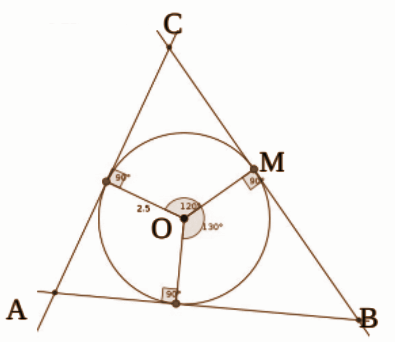Steps of construction:

• Draw a circle of radius 2.5cm with centre O.
• Draw OM as the radius.
• Construct ∠MON = 1200 and ∠MOP = 1300.
• Draw the tangents through M, N and P respectively and meet at A, B and C.
• ∆ABC is the required triangle.

Question 26: A boy saw the top of a building under construction at an elevation of 30o. The completed building was 12m higher and the boy saw its top at an elevation of 60o from the same spot.

[a] Draw a rough figure based on the given details.

[b] What is the height of the building?

[c] What is the distance between the building and the boy?

Solution:

[a]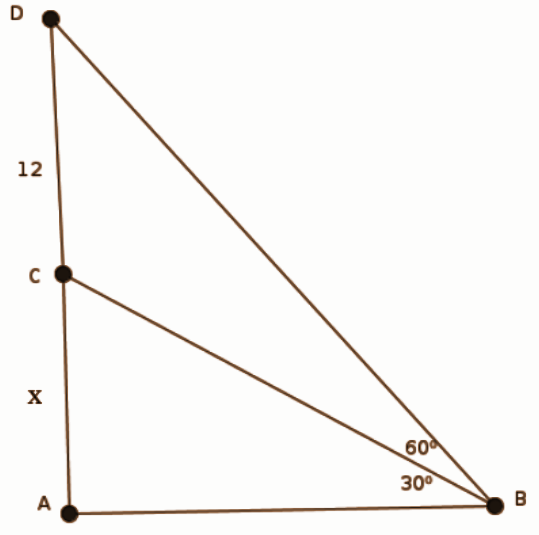[b] Consider AC = x , AD = x + 12.

In triangle ABD, AD / AB = tan 600

AB = AD / tan 600

⇒ AB = x + 12 / √3 …………. (1)

In triangle ABC,

AC / AB = tan 300

⇒ AB = AC / tan 300

⇒ AB = x / [1 / √3]

= x √3 ………. (2)

Comparing equations (1) and (2),

[x + 12] / [√3] = x √3

⇒ 3x = x + 12

⇒ 3x – x = 12

⇒ 2x = 12

x = 6

Hence, the height of the building = 6 + 12 = 18 m.

[c] Consider the equation (2), AB = √3 x

= √3 × 6

= 1. 73 × 6

= 10.38m

Distance between the building and the boy = 10.38m.

Question 27: The picture shows the shape of a boiler. The total height of the boiler is 12m and the diameter is 6m, the height of the cylindrical part is 6m.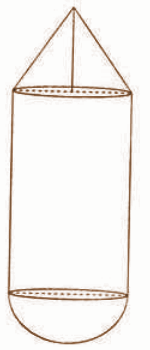[a] What is the height of the cone?

[b] How many litres can the boiler hold?

Solution:

Given, total height = 12m

Radius = 6 / 2 = 3m

Height of the cylinder part (h) = 6m

[a] Height of the cone = 12 – (6 + 3) = 3m.

[b] Volume of the boiler = Volume of the cone + volume of the cylinder + volume of the hemisphere.

[1 / 3] πr2h + πr2h + [2 / 3] πr3

= [1 / 3] × π × 3 × 3 × 3 + π × 3 × 3 × 6 + [2 / 3] × 3 × 3 × 3

= 9π + 54π + 18 π

= 81π m3

= 81 × 3.14

= 254.34 m3

Hence, the volume in liters = 254.34 × 1000 = 254340 liters.

Question 28: A circle with centre (3, 4) passes through the origin.

[a] What is the radius of the circle?

[b] If a point on the circle is (x, y), write the relation between x, y.

[c] Check whether the point (-2, 1) lies on this circle.

Solution: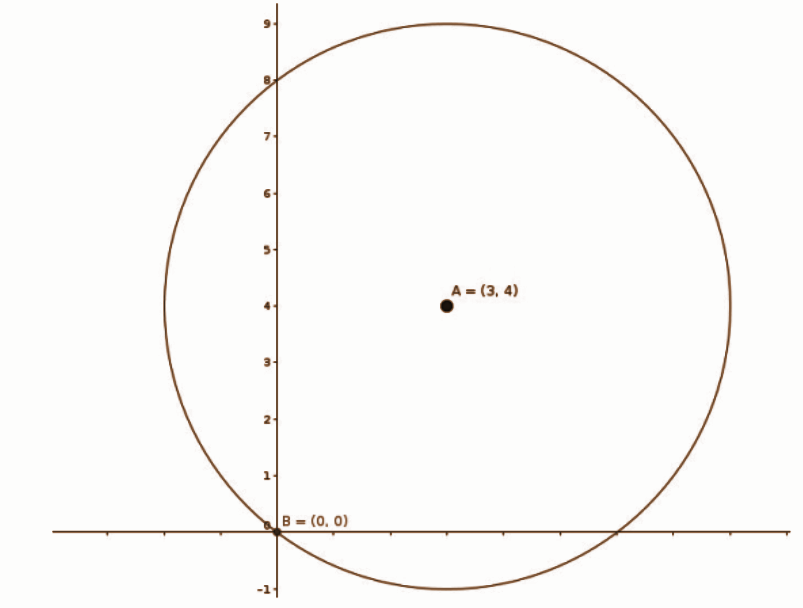Given , center (3, 4) ; Origin (0, 0).

[a] Radius (r) = √x2 + y2

= √32 + 42

= √9 + 16

= √25

= 5

[b] Equation of the circle = (x − a)2 + (y − b)2 = r2

(x − 3)2 + (y − 4)2 = 52

⇒ x2 − 6x + 9 + y2 −8y + 16 = 25

⇒ x2 + y2 −6x − 8y + 25 − 25 = 0

⇒ x2 + y2 − 6x − 8y = 0 is the equation of the circle.

[c] (- 2, 1) , substitute this value in the equation of the circle, we get,

(−2)2 + (1)2 − 6 × −2 −8 × 1 = 0

4 + 1 + 12 – 8 = 0

17 – 8 = 0

9 ≠ 0

AC > radius of the circle.

Hence, the point (-2, 1) lies outside the circle.

Question 29: Read the following, understand the mathematical idea expressed in it and answer the questions that follow.

1, 4, 9, 16 …… are the squares of the counting numbers. The remainders got by dividing the square numbers with natural numbers have a cyclic property. For example, the remainder on dividing these numbers by 4 are tabulated here.

 Number 1 4 9 16 25 – – – Remainder 1 0 1 0 1 – – –

On dividing by 4 perfect squares leave only 0 and 1 as remainders. From this, we can conclude that an arithmetic sequence whose terms leaves remainder 2 on dividing by 4 does not have a perfect square.

[a] Which are the possible remainders on dividing any number with 4?

[b] Which are the numbers we would not get on dividing a perfect square by 4?

[c] What is the remainder that leaves on dividing the terms of the arithmetic sequence 2, 5, 8, 11, ………… by 4?

[d] Does the arithmetic sequence 3,7,11,…………..contain perfect squares?

[e] Write a sequence with common difference 4 which contains many perfect squares.

Solution:

[a] 0, 1, 2 and 3

[b] 2 and 3

[c] 2, 1, 0 and 3

[d] No, the sequence doesn’t contain perfect square numbers.

3, 7, 11, … …… is divided by 4 we get the remainder 3. When divided by the perfect square numbers by 4, we get the remainder 0 and 1.

[e] 1, 5, 9, 13 ……………